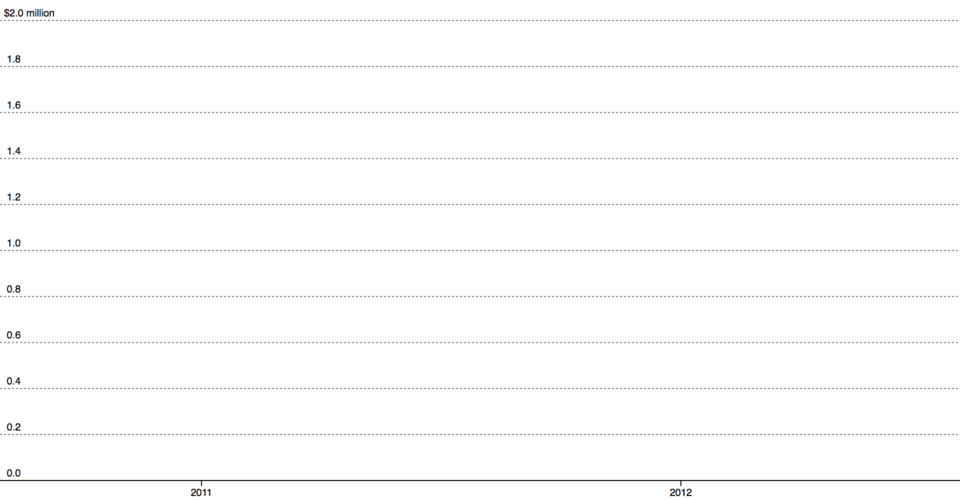# # d3-axis

## # Installing

``````<script src="https://d3js.org/d3-axis.v1.min.js"></script>
<script>

var axis = d3.axisLeft(scale);

</script>
``````

## # API Reference

``````d3.select("body").append("svg")
.attr("width", 1440)
.attr("height", 30)
.append("g")
.attr("transform", "translate(0,30)")
.call(axis);
``````(opens new window)

``````<g fill="none" font-size="10" font-family="sans-serif" text-anchor="middle">
<path class="domain" stroke="#000" d="M0.5,6V0.5H880.5V6"></path>
<g class="tick" opacity="1" transform="translate(0.5,0)">
<line stroke="#000" y2="6"></line>
<text fill="#000" y="9" dy="0.71em">0.0</text>
</g>
<g class="tick" opacity="1" transform="translate(176.5,0)">
<line stroke="#000" y2="6"></line>
<text fill="#000" y="9" dy="0.71em">0.2</text>
</g>
<g class="tick" opacity="1" transform="translate(352.5,0)">
<line stroke="#000" y2="6"></line>
<text fill="#000" y="9" dy="0.71em">0.4</text>
</g>
<g class="tick" opacity="1" transform="translate(528.5,0)">
<line stroke="#000" y2="6"></line>
<text fill="#000" y="9" dy="0.71em">0.6</text>
</g>
<g class="tick" opacity="1" transform="translate(704.5,0)">
<line stroke="#000" y2="6"></line>
<text fill="#000" y="9" dy="0.71em">0.8</text>
</g>
<g class="tick" opacity="1" transform="translate(880.5,0)">
<line stroke="#000" y2="6"></line>
<text fill="#000" y="9" dy="0.71em">1.0</text>
</g>
</g>
``````

# d3.axisTop(scale) <源码> (opens new window)

# d3.axisRight(scale) <源码> (opens new window)

# d3.axisBottom(scale) <源码> (opens new window)

# d3.axisLeft(scale) <源码> (opens new window)

# axis(context) <源码> (opens new window)

# axis.scale([scale]) <源码> (opens new window)

# axis.ticks(arguments…) <源码> (opens new window)
# axis.ticks([count[, specifier]])
# axis.ticks([interval[, specifier]])

``````axis.ticks(20, "s");
``````

``````axis.ticks(d3.timeMinute.every(15));
``````

``````axis.ticks(10);
``````

``````axis.tickArguments();
``````

# axis.tickArguments([arguments]) <源码> (opens new window)

``````axis.tickArguments([20, "s"]);
``````

``````axis.tickArguments([d3.timeMinute.every(15)]);
``````

# axis.tickValues([values]) <源码> (opens new window)

``````var xAxis = d3.axisBottom(x)
.tickValues([1, 2, 3, 5, 8, 13, 21]);
``````

# axis.tickFormat([format]) <源码> (opens new window)

``````axis.tickFormat(d3.format(",.0f"));
``````

``````axis.ticks(10, ",f");
``````

# axis.tickSize([size]) <源码> (opens new window)

# axis.tickSizeInner([size]) <源码> (opens new window)

# axis.tickSizeOuter([size]) <源码> (opens new window)